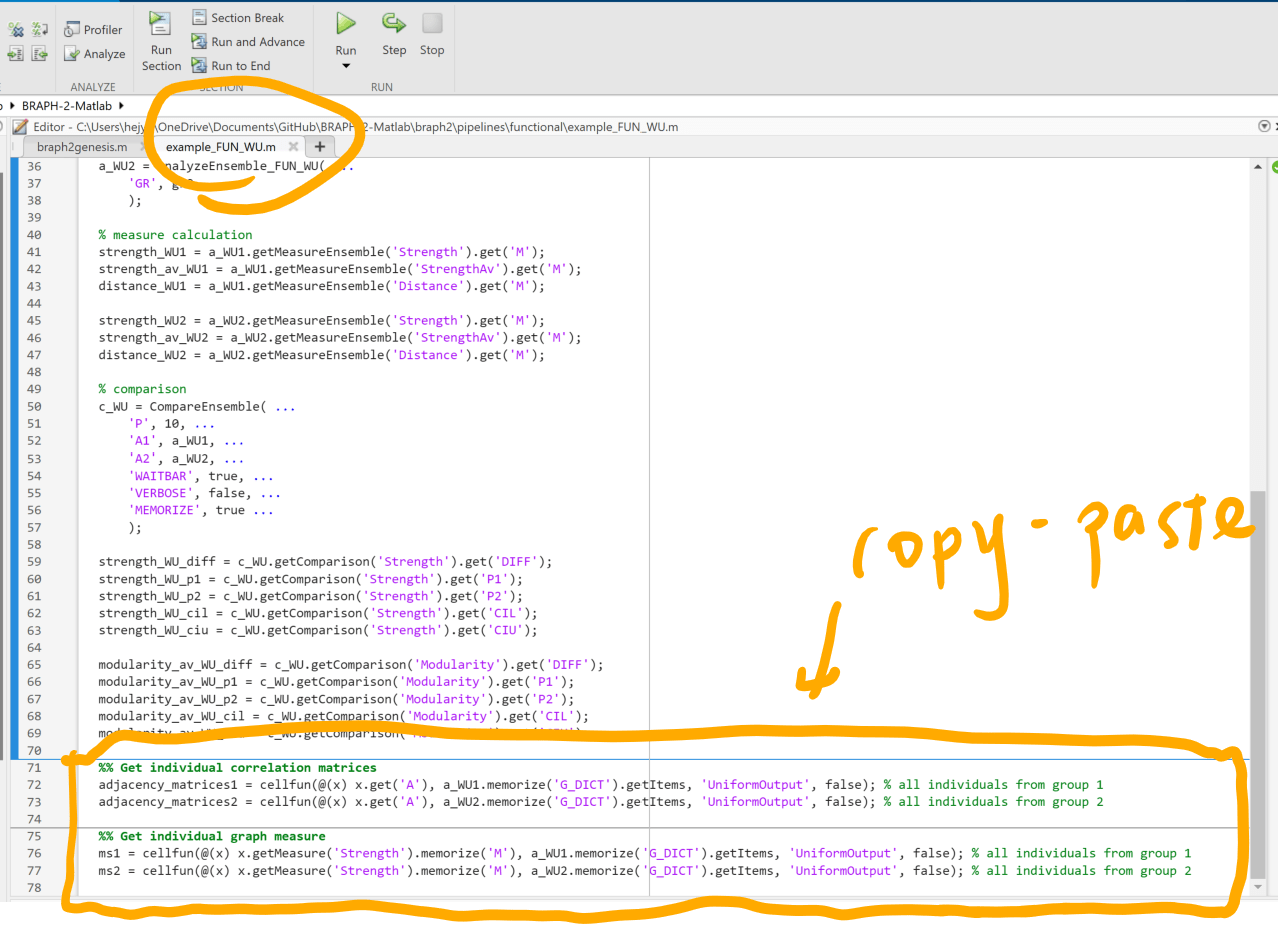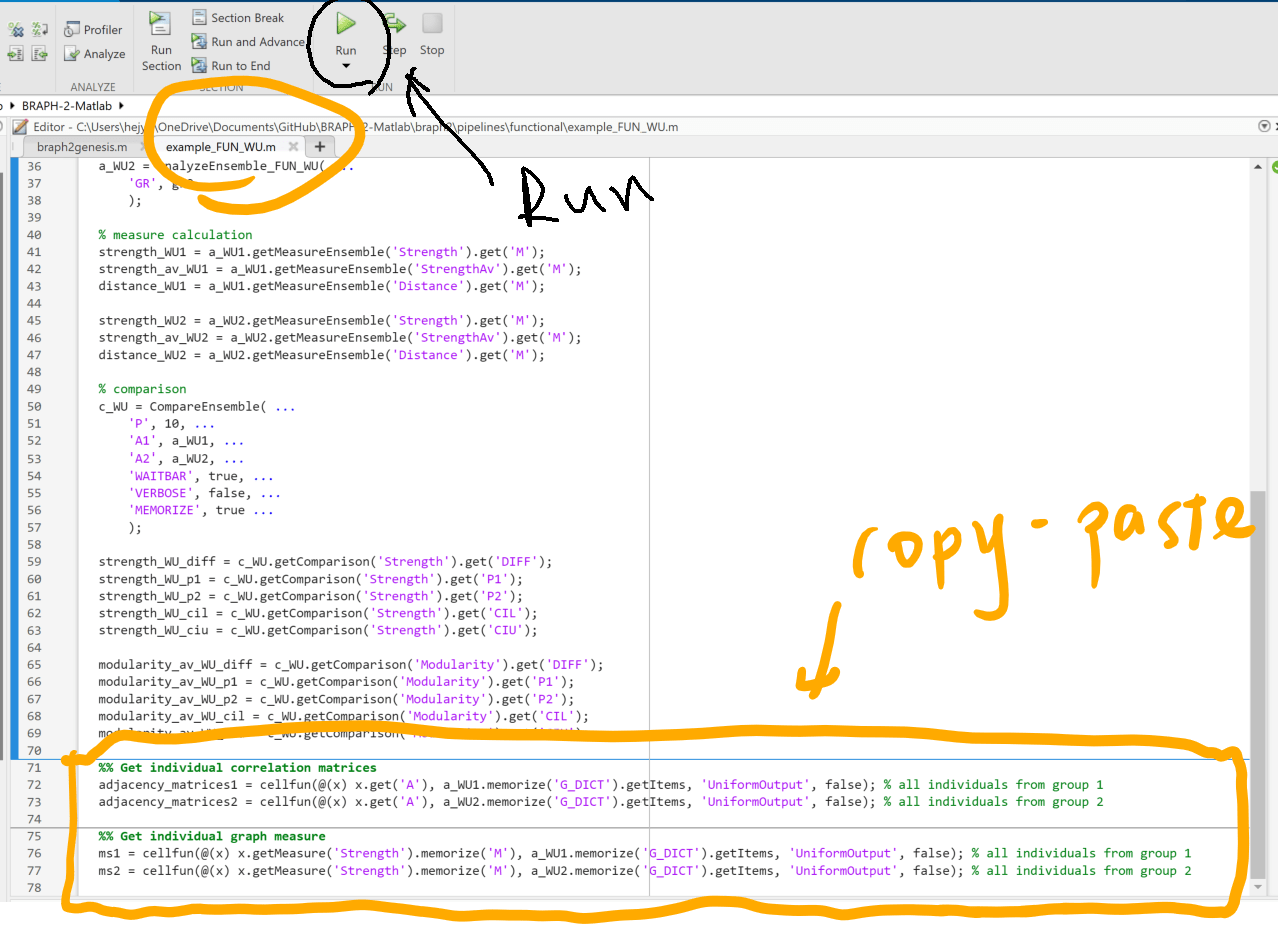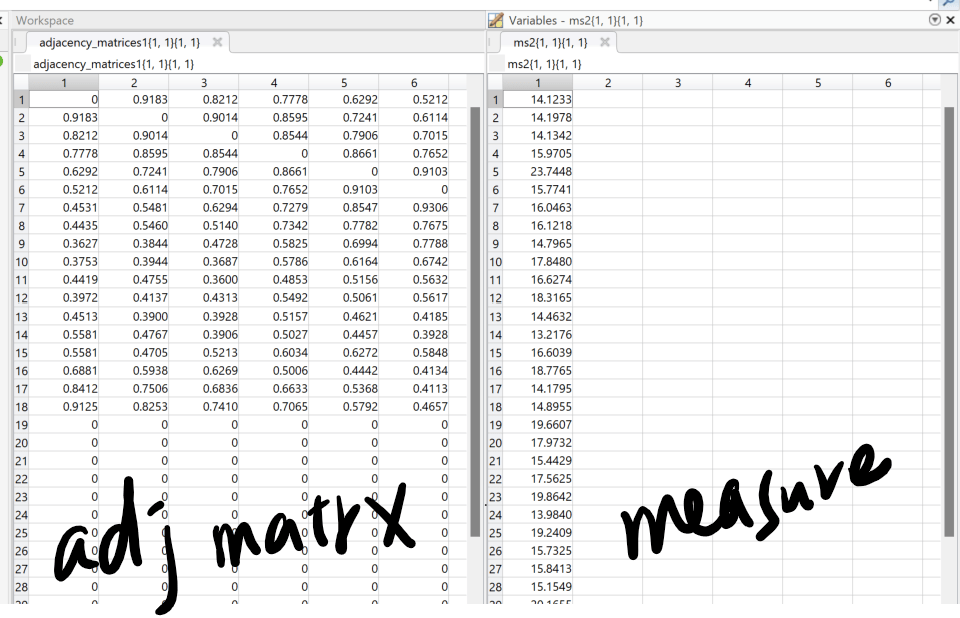# Reply To: Export graph metric values

Home Forums General Help (Braph 2.0) Export graph metric values Reply To: Export graph metric values

#173810

Hi Sai Prasanna,

Thanks for the follow-up feedback.
I would like to provide further information based on example_FUN_WU.m about the following:
(1) Get individual graph metrics/graph measures.
(2) Get individual correlation matrices/adjacency matrices.

Step 1: Copy and paste the following codes at the end of example_FUN_WU.m

``````%% Get individual correlation matrices
adjacency_matrices1 = cellfun(@(x) x.get('A'), a_WU1.memorize('G_DICT').getItems, 'UniformOutput', false); % all individuals from group 1
adjacency_matrices2 = cellfun(@(x) x.get('A'), a_WU2.memorize('G_DICT').getItems, 'UniformOutput', false); % all individuals from group 2

%% Get individual graph measure
ms1 = cellfun(@(x) x.getMeasure('Strength').memorize('M'), a_WU1.memorize('G_DICT').getItems, 'UniformOutput', false); % all individuals from group 1
ms2 = cellfun(@(x) x.getMeasure('Strength').memorize('M'), a_WU2.memorize('G_DICT').getItems, 'UniformOutput', false); % all individuals from group 2``````Step 2: Run the revised example_FUN_WU.m by clicking on the run buttonStep 3: Check the variable adjacency_matrices1 for all individual correlation matrices (weighted-undirected in this example) from group 1, and adjacency_matrices2 for group 2

Step 4: Check the variable ms1 for all individual calculated metrics (strength in this example) from group 1, and ms2 for group 2Thank you, and let me know if you have any questions.
Best regards,
Yu-Wei